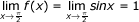# Engineering Maths - Calculus

>>>>>>>>Calculus

• A

x = ky• B

kx = y• C

x = y• D

None of these• A

f(a) is an extreme value of f(x) if f '(a)=0• B

If f(a) is an extreme value of f(x), then f '(a) = 0• C

If f '(a) = 0, then f (a) is an extreme value of f(x)• D

all of these• A

f'(0) = 0• B

f(x) is maximum at x = 0• C

f(x) is minimum at x = 0• D

None of these• A

x= 3 and x = 2• B

x = 1 and x = 3• C

x = 2 and x = 3• D

x = 3 and x = 4• Option : C
• Explanation : f(x)=2x3-15x2+36x+10 f '(x)=6x2-30x+36 and f ''(x)=12x-30 For maxima and minima, f '(x)=0 6x2-30x+36 = 0 or x2- 5x + 6 = 0 or X = 3,2
Putting these values of x in equation (ii), we get
f '(3) = 36 - 30 = + 6 positive
Hence minimum value = 3
f ' (2) = 24 - 30 = - 6 negative, hence maximum value = 2

• A

2π/3• B

π/2• C

π/3• D

1• Option : D
• Explanation :Related Quiz.
Calculus